Question
Part b & c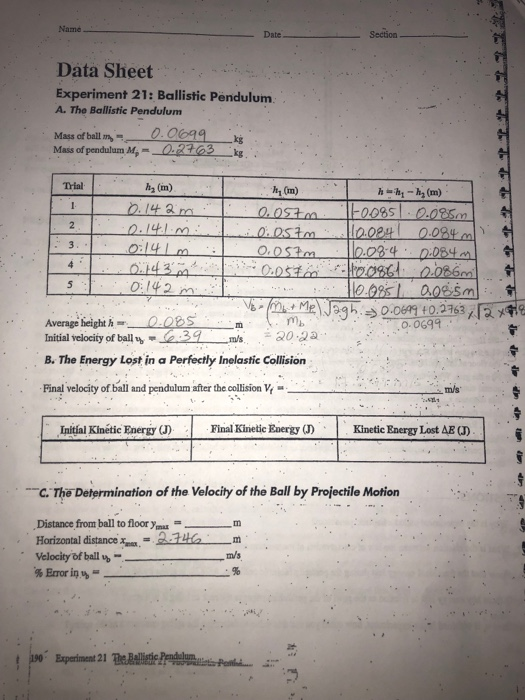The given problem based on perfectly inelastic collision. To solve this problem we use law of conservation of linear momentum and law of conservation of energy.

NOTE: In part c u forget to note the value of Ymax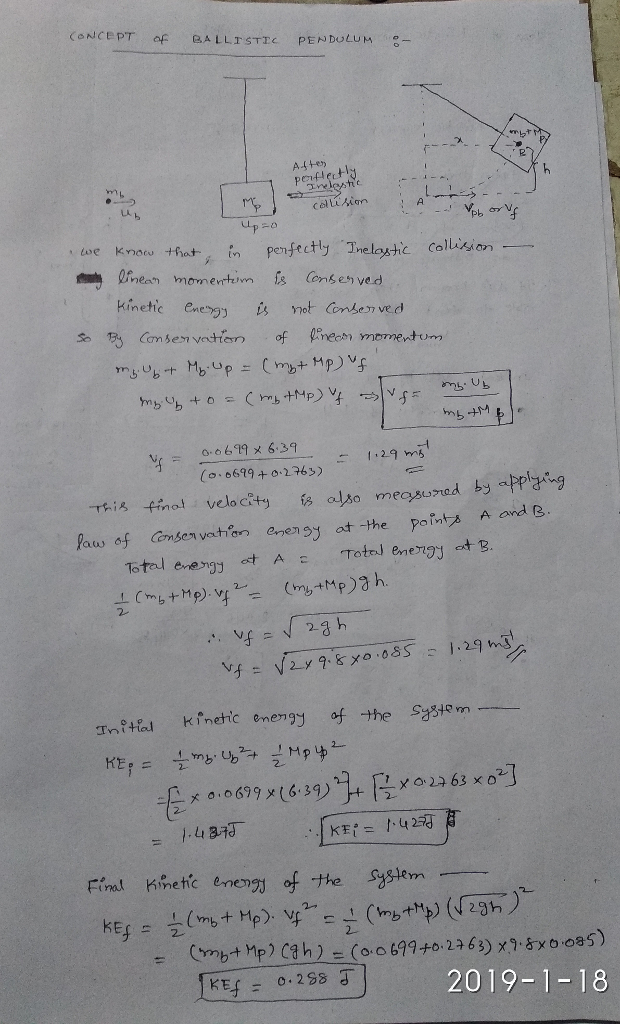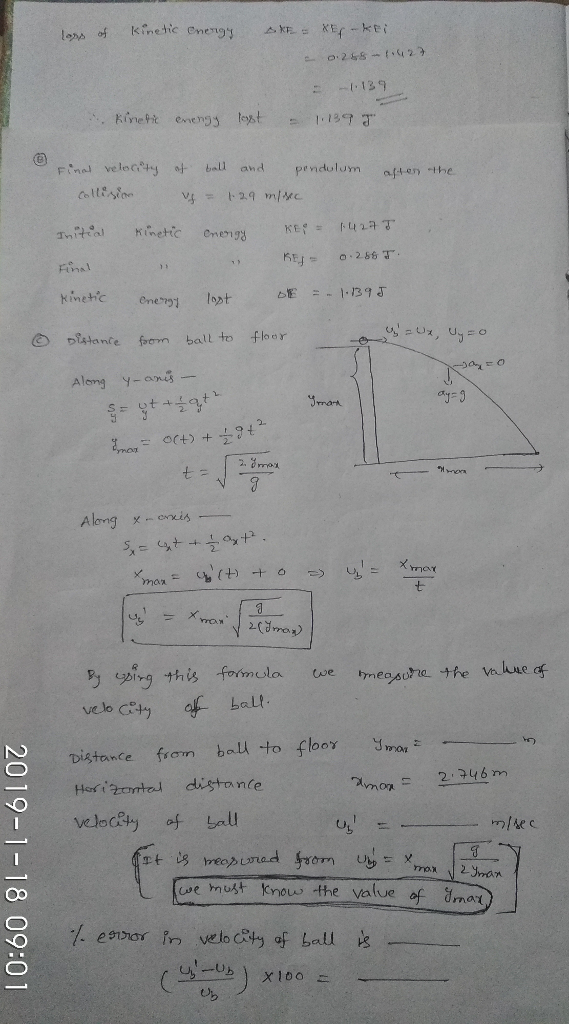#### Earn Coins

Coins can be redeemed for fabulous gifts.

Similar Homework Help Questions
• ### Name Date Section Data Sheet Experiment 21: Ballistic Pendulum. A. The Ballistic Pendulum Mass ofball m-9.890....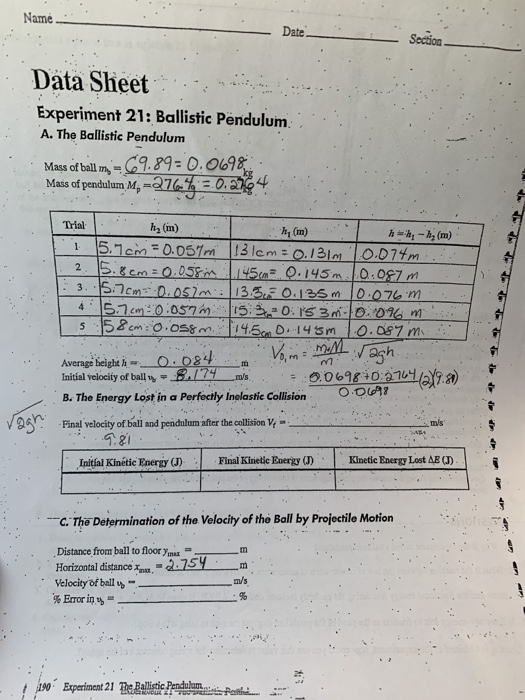Name Date Section Data Sheet Experiment 21: Ballistic Pendulum. A. The Ballistic Pendulum Mass ofball m-9.890. 0698 Mass of pendulum Mp- Trial h2 (m) hy (m) 4.1らコcm : 0.057 in 115.220. IS 3m-10.01am Average beight h Initial velocity of ballm's B. The Energy Lost in a Perfocily Inolastic Collsion O 00 698 01a nal velodic mis Initíal Kinétic Energy (J) Final Kinetic EnergyKinetic Energy Lost AB C. The Determination of the Velocity of the Ball by Projectile Motion Distance from...

• ### In the ballistic pendulum experiment (A.K.A conservation of momentum & energy), the velocity of the projectile...

In the ballistic pendulum experiment (A.K.A conservation of momentum & energy), the velocity of the projectile was measured and recorded (vo = 5 m/s). The mass of the projectile was 12 g and the ballistic pendulum mass was 148 g. After the projectile is fired, it hits the ballistic pendulum, and both moved with velocity. Calculate the velocity LaTeX: vv of the projectile and the pendulum after the collision.

• ### I put the theory on first page. 7-Ballistic Pendulum Data Studio File: "Ballistie Pendlds" Equipment List...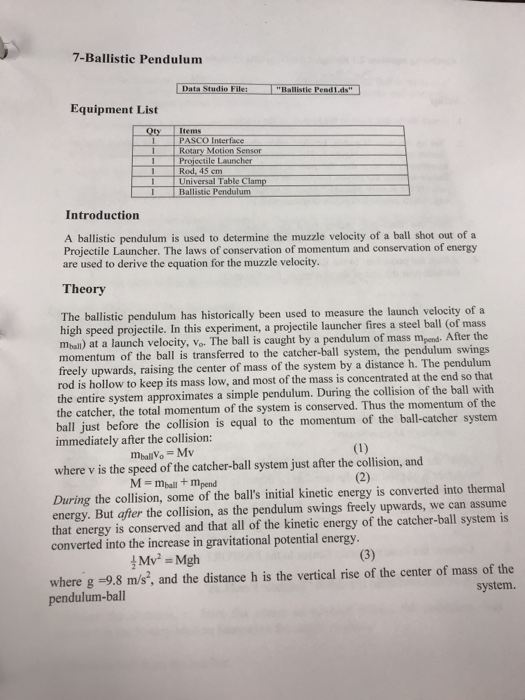I put the theory on first page. 7-Ballistic Pendulum Data Studio File: "Ballistie Pendlds" Equipment List Qty Items PASCO Interface Rotary Motion Sensor Rod, 45 em Ballistic Pendulum 1Universal Table Clamp Introduction A ballistic pendulum is used to determine the muzzle velocity of a ball shot out of a Projectile Launcher. The laws of conservation of momentum and conservation of energy are used to derive the equation for the muzzle velocity. Theory The ballistic pendulum has historically been used to...

• ### Virtual Lab: Ballistic Pendulum Introduction The ballistic pendulum was invented by Benjamin Robins in 1742 as...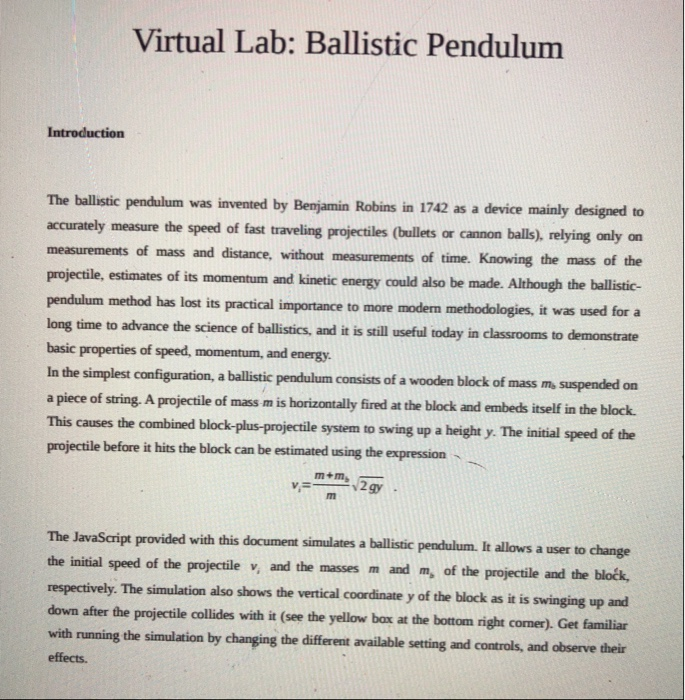Virtual Lab: Ballistic Pendulum Introduction The ballistic pendulum was invented by Benjamin Robins in 1742 as a device mainly designed to accurately measure the speed of fast traveling projectiles (bullets or cannon balls), relying only on measurements of mass and distance, without measurements of time. Knowing the mass of the projectile, estimates of its momentum and kinetic energy could also be made. Although the ballistic- pendulum method has lost its practical importance to more modern methodologies, it was used for...

• ### Consider a projectile (0.1 kg) fired into a ballistic pendulum (0.5 kg, r_cm = 0.3 m),...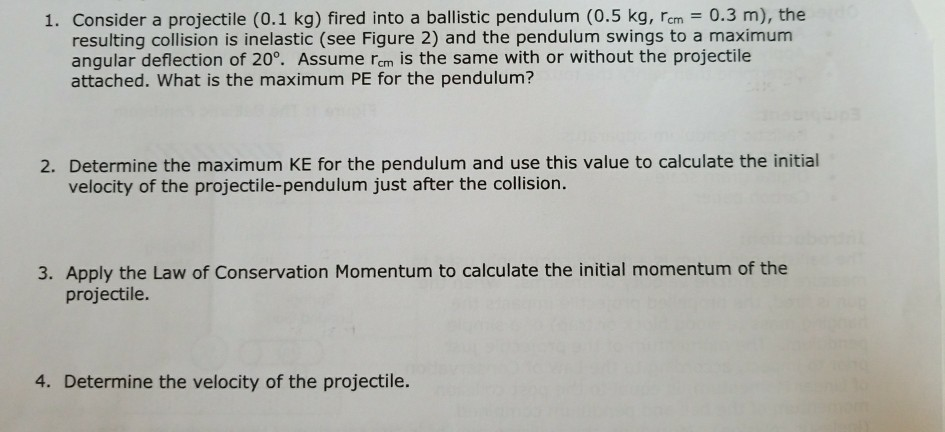Consider a projectile (0.1 kg) fired into a ballistic pendulum (0.5 kg, r_cm = 0.3 m), the resulting collision is inelastic (see Figure 2) and the pendulum swings to a maximum angular deflection of 20 degree. Assume r_cm is the same with or without the projectile attached. What is the maximum PE for the pendulum? Determine the maximum KE for the pendulum and use this value to calculate the initial velocity of the projectile-pendulum just after the collision. Apply the...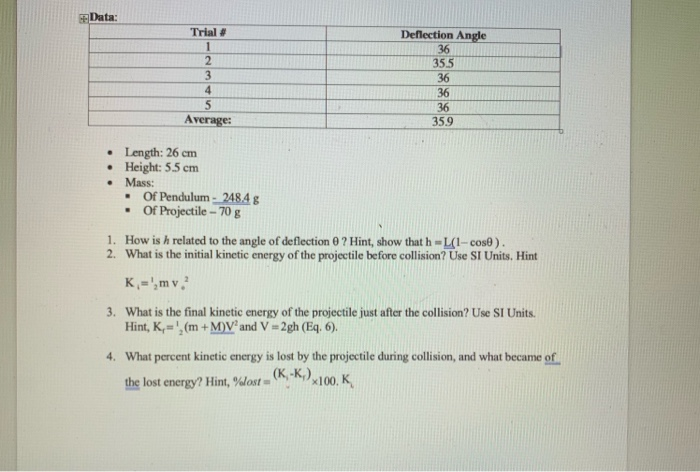Please help me answer the 4 questions at the bottom for my ballistic pendulum lab. Thank you! Data: Trial # Deflection Angle 36 35.5 36 36 36 35.9 2 Average: Length: 26 cm Height: 5.5 cm Mass: .Of Pendulum- 248.4g Of Projectile-70 g 1. 2. How is h related to the angle of deflection θ ? Hint, show that h-Lil-cod) What is the initial kinetic energy of the projectile before collision? Use SI Units. Hint 3. What is the final...

• ### Need help With Analysis questions. Ballistic Pendulum Lab In this lab, you will explore conservat...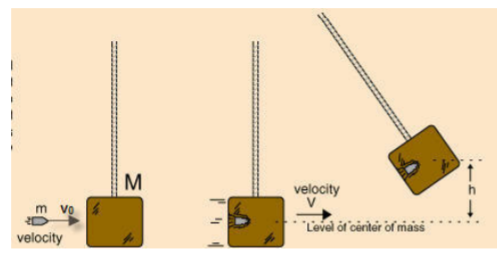Need help With Analysis questions. Ballistic Pendulum Lab In this lab, you will explore conservation of Energy and Momentum in an inelastic collision between a projectile and a cardboard box suspended from a string. In particular, you will see that the transfer of linear momentum is easily accounted for in an inelastic collision, but the transfer of kinetic energy is not easy to measure directly. This is because Kinetic Energy in collisions transfers to vibrational, acoustic, and potential energy. Once...

• ### Derive the expression for the initial velocity v of a ballistic pendulum in terms of the...

Derive the expression for the initial velocity v of a ballistic pendulum in terms of the swept-out angle theta. Use the following equations of momentum and energy conservation... When the projectile and the pendulum stick together, they have total mass m + M and we define their combined velocity to be V⃗ . The relationship from conservation of momentum is: mv = (m + M)V⃗ Conservation of energy requires the initial kinetic energy of the pendulum system to equal the...

• ### Ballistic Pendulum Version Procedure: Fire a practice shot. Locate the landing point on the floor then...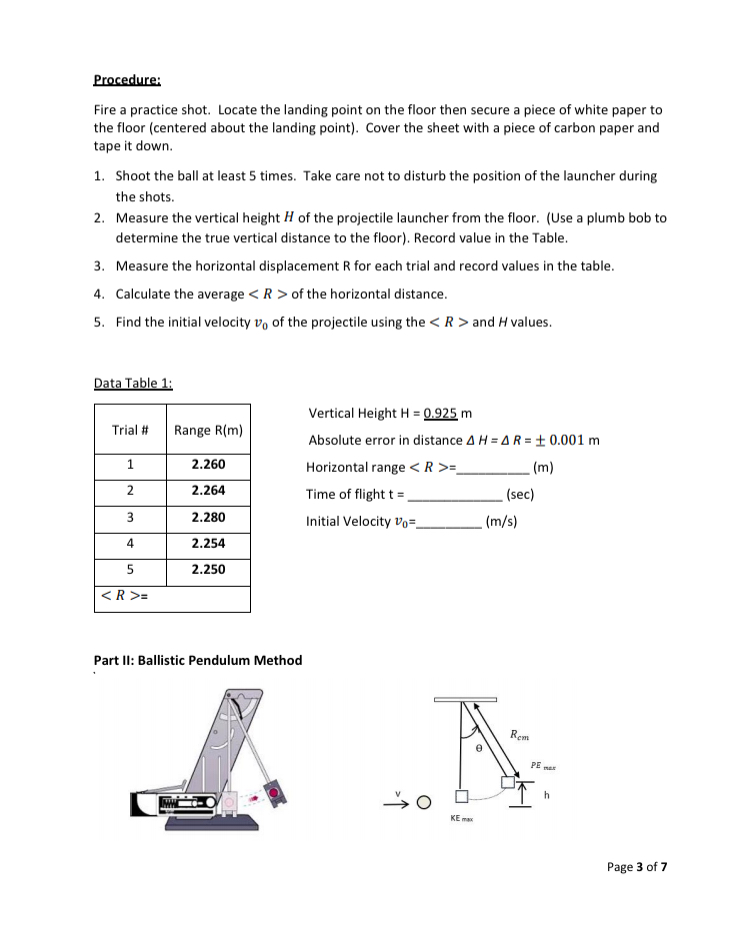Ballistic Pendulum Version Procedure: Fire a practice shot. Locate the landing point on the floor then secure a piece of white paper to the floor (centered about the landing point). Cover the sheet with a piece of carbon paper and tape it down. 1. Shoot the ball at least 5 times. Take care not to disturb the position of the launcher during the shots. 2. Measure the vertical height H of the projectile launcher from the floor. (Use a plumb...

• ### A bullet of mass 8 g is fired into a 1.2 kg ballistic pendulum (a block...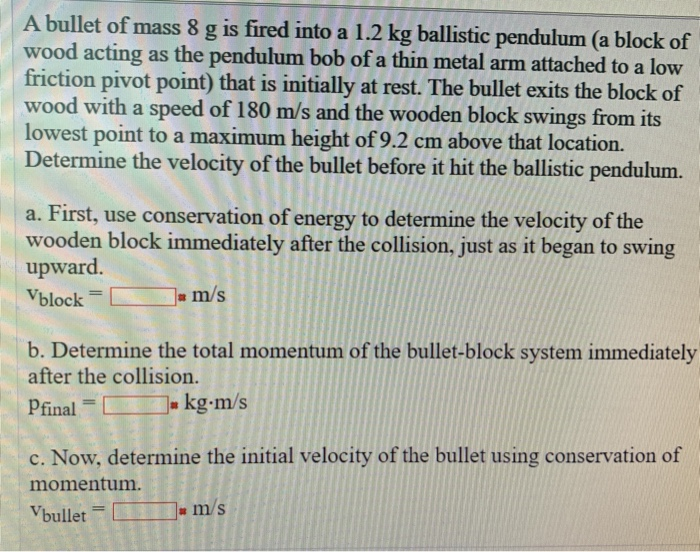A bullet of mass 8 g is fired into a 1.2 kg ballistic pendulum (a block of wood acting as the pendulum bob of a thin metal arm attached to a low friction pivot point) that is initially at rest. The bullet exits the block of wood with a speed of 180 m/s and the wooden block swings from its lowest point to a maximum height of 9.2 cm above that location. Determine the velocity of the bullet before it...